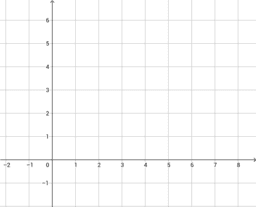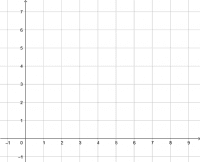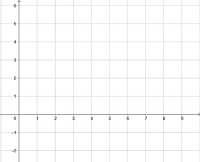# Properties of a Circle Activity

Topic:
Circle
Students will be able to solve problems and justify the solution strategy, using the following circle properties: a. The perpendicular from the centre of a circle to a chord bisects the chord. b. The measure of the central angle is equal to twice the measure of the inscribed angle subtended by the same arc. c. The inscribed angles subtended by the same arc are congruent. d. A tangent to a circle is perpendicular to the radius at the point of tangency. Provide an example of each property -Solve a given problem involving application of one or more of the circle properties. -Determine the measure of a given angle inscribed in a semicircle, using the circle properties. -Explain the relationship among the centre of a circle, a chord and the perpendicular bisector of the chord.
•### Circle Part 1

•### Circle Part 2

•### Circle Part 3

•